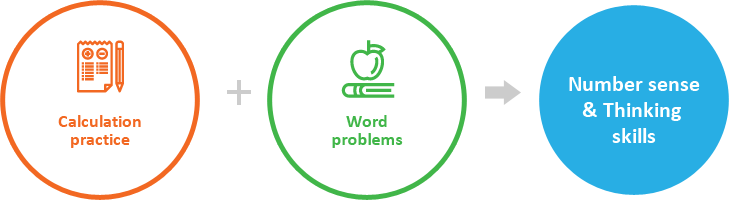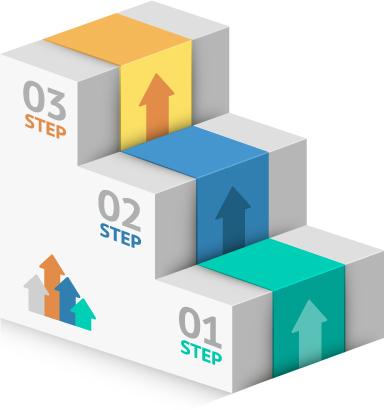바로가기 메뉴
컨텐츠바로가기
주메뉴바로가기
하단메뉴바로가기

• Curriculum
• Resources
• Contact

# The Tiger Math Approach

In Tiger Math, kids understand the math concepts first, practice repeatedly, and then apply it to a word problem.In Tiger Math, kids build up number sense and mathematical thinking ability by doing calculation practice and word problems on a daily basis.In Tiger Math, kids learn math in a step-by-step approach.
During 80% of daily math practice,

kids repeat the concept they have learned through previous sessions, and during 20% of daily math practice, they learn new math concepts.

This way, children can become more comfortable through
reinforcement gained through practice and can start exploring newer concepts so that they can reach their maximum capacity.